Courses

# Chapter 3 - Laws of Motion JEE Notes | EduRev

## JEE : Chapter 3 - Laws of Motion JEE Notes | EduRev

The document Chapter 3 - Laws of Motion JEE Notes | EduRev is a part of JEE category.
All you need of JEE at this link: JEE

• Newton’s first law : Every body continues in its state of rest or of uniform motion in a straight line unless compelled by some external force to change that state.
• Newton’s first law is called law of Inertia. The principle of inertia and the foundation of the first law were laid down by Galileo. Inertia is that property of body owing to which the body opposes any change in its state of motion.
• Inertia of rest is the inability of body to change its state of rest by itself, while inertia of motion is the inability of the body to change its state of motion by itself.
• Inertia of direction is the inability of the body to change its direction of motion by itself.
• Newton’s first law of motion gives the definition of force and the concept of inertia.
• Physical independence of force is a consequence of first law of motion.
• The quantity of motion possessed by a body is called momentum. It is measured by the product of mass and velocity.
• Newton’s second law says that the rate of change of momentum is directly proportional to the applied force.
• An accelerated motion is the result of application of the force.
• Acceleration produced in the body depends only on its mass and not on the final or initial velocity.
• Newton’s second law of motion gives the idea about the measurement of force.
• Force is an external effort in the form of push or pull which (i) one produces or tries to produce motion in a body at rest, or (ii) stops or tries to stop a moving body, or (iii) changes or tries to change the direction of motion of the body.
• For simplicity, all forces between objects can be placed into two broad categories - (a) contact forces and (b) forces resulting from action at a distance.
• Frictional force, tensional force, normal force, air resistance force, applied force and spring force are contact forces.
• Gravitational force, electrical force, weak force are action-at-a distance forces.
• The relative strengths of the gravitational force, the weak force, the electromagnetic force and the strong nuclear force are
Fg : Fw : Fe : F: : 1 : 1025 : 1036 : 1038
• The gravitational force is the force of attraction between two masses. It can be extended upto infinity. It is a central force and conservative in nature. It is the weakest force.
• The force between two static charges is called electrostatic force.
• The force between two magnetic poles is called magnetic force.
• If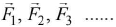are the concurrent forces acting on the same point, then the point will be in equilibrium if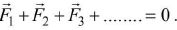• When many forces act on a body simultaneously at its different points but all in same plane, then they are called coplanar forces.
• Impulse is the measure of the degree to which an external force produces a change in momentum of the body The product of a large force acting on a body for a small interval is called impulse.
• Newton’s third law of motion says that every action has equal and opposite reaction. Action and reaction act on different bodies and they are simultaneous.
• Action and reaction never cancel each other.
• The speed of driving a car safely in darkness depends upon range of the headlight.
• Newton’s first and third laws can be derived from the second law therefore second law is the most fundamental law out of the three laws.
• The law of conservation of linear momentum is a logical consequence of Newton’s second law.
• If n bullets each of mass m and velocity v are fired from a gun then the average force acting on the gun is mnv.
• Principle of conservation of momentum follows from Newton’s first law of motion.
• Newton’s third law contains law of conservation of momentum.
• Swimming becomes possible because of third law of motion.
• In case of a uniform circular motion, the change in momentum with time is not zero,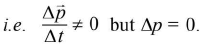• Those frames which are at rest with respect to one another or which are moving with uniform speed with respect to one another are called inertial reference frames.
• Newton’s laws of motion are valid only in inertial frame of reference.
• A reference frame attached to the earth is an inertial frame by definition.
• When the lift is at rest or in uniform motion, then the weight recorded by the spring balance is equal to the actual weight of the body.
• When the lift is accelerated up, then the weight recorded by the spring balance is more than the actual weight of the body.
• When the lift is accelerated down, the weight recorded by the spring balance is less than the actual weight of the body.
• For a lift falling freely, acceleration of the lift is g and hence the body will feel weightlessness.
• The spring balance will record an increase in weight for a moment, when the lift starts to move up with uniform velocity and then will record the actual weight of the body.
• When the lift just begins to move down with uniform speed, the spring balance will record a decrease in weight for a moment and then will record the actual weight of the body.
• If the downward acceleration of the lift is greater than g, then IV is negative. This means that the body will be accelerated upto the ceiling of the lift.
• If no external force acts on a system of two or more bodies, then the total momentum of the system remains constant. This is known as the principle of conservation of momentum.
• The motion of a rocket is based on principle of conservation of momentum.
• Thrust on the rocket at any instant is equal to the product of the exhaust speed of the burnt gases and the rate of combustion of fuel at that instant.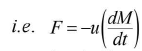-ve sign indicates that thrust on the rocket is in a direction opposite to the direction of escaping gas.
• Velocity of rocket at any instant t, when mass of the rocket is M, is given by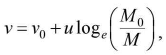where v0 is the initial velocity of the rocket.
• If the initial velocity of rocket is zero, then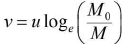If effect of gravity is also taken into account, then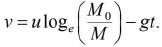Friction

• Force of friction opposes relative motion of the point of contact with respect to the surface.
• Force of friction acts parallel to the surface.
• The number of frictional forces acting on a body depends upon the number of contact surfaces. For every contact surface, there is a frictional force.
• Frictional forces are produced on account of intermolecular interactions between the two bodies or surfaces.
• Frictional force is a self adjusting force which increases with increasing applied force till the body is at rest but on the point of motion.
• For frictional force relative motion between two bodies or surfaces is not necessary. In fact, contact between two bodies or surfaces is necessary.

Types of frictional forces

• Static friction : It is a self-adjusting force with an upper limit called the limiting friction.
• Dynamic friction/kinetic friction
• Sliding friction
• Rolling friction
• Rolling friction < dynamic friction < sliding friction
• If μs = static coeffrcient of friction, R = N = normal reaction, then static frictional force < μsR (body is at rest). Limiting friction = μsR (body is on point of motion).
• If μk= kinetic coefficient of friction, then kinetic frictional force = μkR (body is in motion).
• The limiting frictional force between two surfaces in contact with each other depends only on μ (nature of surfaces) and normal contact force R. It does not depend on their shape, size or surface area.
• Value of μ is always less than one.
• μs = tan λ where λ denotes angle of friction.
• The value of angle of friction for perfectly smooth surface is zero.
• Angle of friction = angle of repose (which is the angle of plane such that a body lying on it is on point of sliding down the plane).

Tension

• Tension force always pulls a body.
• Tension can never push a body or rope.
• Tension across a massless pulley or frictionless pulley remains constant.
• Rope becomes slack when tension force becomes zero.
• When a rope is pulled by a force, the rope becomes tight or taut. This conveys the concept of tension.
• Tension is a reactive force. It is not an active force. A tension cannot be applied externally.

Contact force - Motion of bodies in contact

(a) Two bodies in contact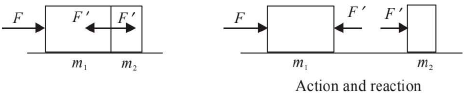A force F is applied on mass m, horizontally. Masses m1 and m2 are in contact. Contact force between m1 and m2 = F' . Contact force is that force with which one body presses the other at the point of contact when the two bodies are placed on a frictionless surface.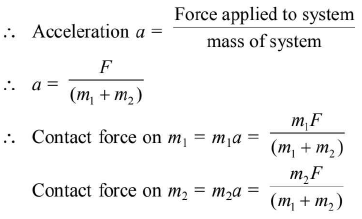or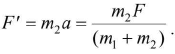(b) Three bodies in contact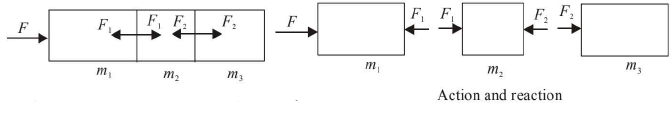Force applied on the system = F
Mass of the system = m1 + m2 + m3
Acceleration of the system = a.
∴ Acceleration of each mass (a) =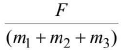Contact force between m1 and m2 = F1
Contact force between mand m3 = F2
For first body, F - F1 = m1a
For second body, F1 - F2 = m2a
For third body, F2 = m3a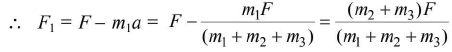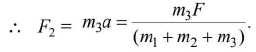(c) Motion of bodies connected by strings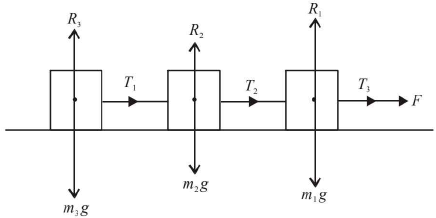Acceleration in system = a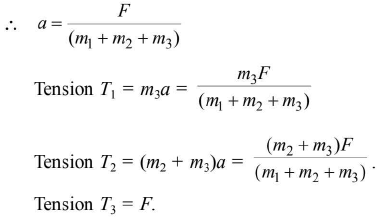Pulley

• Consider two masses m1 and m2 connected at the two ends of a massless inextensible string passing over a ffictionless pulley of mass M.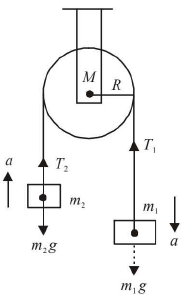Let the mass of pulley = M
Equations of motion are :
m1g - T1 = m1a ... (i)
T2 - m2g = m2a ... (ii)
Consider torque on pulley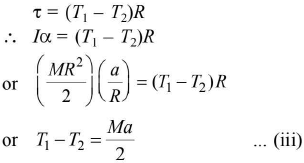From (i), (ii) and (iii) we get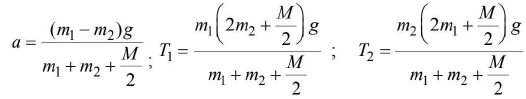Reaction at pulley R = T1 + T2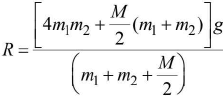- If the mass of the pulley is zero, put M = 0.
- If the two masses are equal, put m1 = m2 = m.
• Consider a body of mass mwhich rests on a surface which is horizontal. Let a string passing over a pulley connect m2 with mass m1 as shown in figure.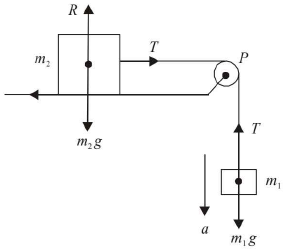(a) Without friction between m2 and horizontal table,
Acceleration, a =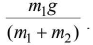Tension, T =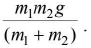(b) With friction between body m2 and table,
Acceleration, a =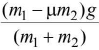Tension, T =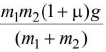• Two masses are suspended as shown in figure.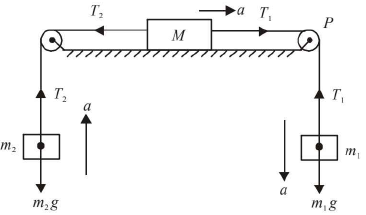The block on horizontal table moves towards right with acceleration a.
m1g - T1 = m1a ... (i)
T2 - m2g = m2a ... (ii)
T1- T2 = Ma ... (iii)
We get, acceleration, a =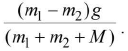• Two masses are suspended over a pulley on an inclined plane as shown in the figure. The mass m1 descends with an acceleration a. Mass m2 is on inclined plane.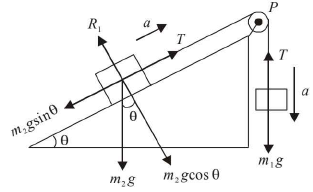Without friction:
Acceleration, a =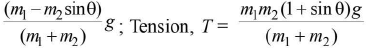With friction: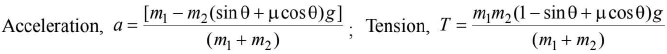• Masses m1 and m2 are connected by a string passing over a pulley such that m1> m2.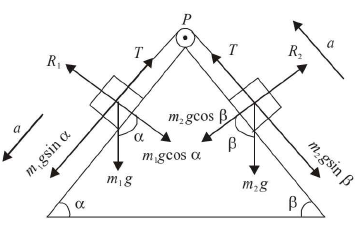Acceleration, a =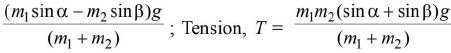• The mass M is connected to m1 and m2 via strings over two light pulleys. Let m1 > m2. Obviously m1 moves down with acceleration a.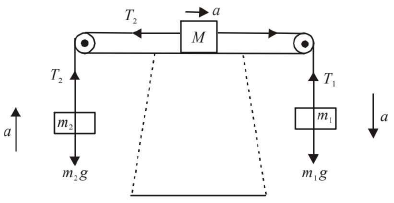The equations are :m1g - T1 = m1a;
T2 - m2g = m2a; T1 - T2 = Ma.
By solving these equations, we get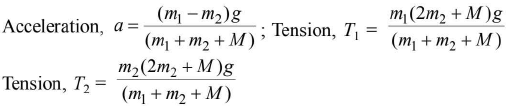Corollaries
• When the two masses are equal,
m1 = m2 = m.
Acceleration = zero
Reaction at pulley = 2T = 2mg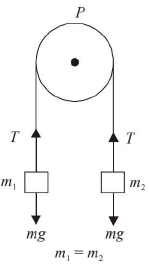(a) What happens if a mass m is hanging at one end of string and a student of equal mass m starts climbing up along the string from other end?By conservation of momentum, the student and the mass will rise up with same speed.
(b) What happens if one student climbs from one end of string and the other of equal mass but faster speed climbs from other end?
The two will reach the pulley simultaneously.
• Motion on an inclined plane
The inclined plane may be rough or smooth. A body may move down the plane or up the plane.
(i) Motion of a body down the rough plane: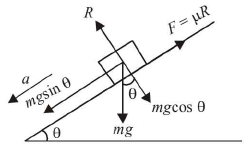Normal reaction R = mgcosθ
mgsinθ - μR = ma
∴ mgsinθ - μmgcosθ = ma
or a = g(sinθ - pcosθ)
∴ Acceleration down the rough plane = g(sinθ - pcosθ).
(ii) Motion of a body down the smooth plane: (μ = 0)
∴ Acceleration of body = gsinθ.
(iii) Motion of a body up the rough plane: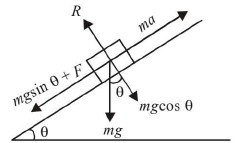When the body moves up the plane, the force of friction F= μR acts down the plane to oppose the motion.
∴ ma = mgsinθ + F = mgsinθ + μR
= mgsinθ + μmgcosθ = mg( sinθ + μcosθ).
or acceleration a = g(sinθ + μcosθ).
(iv) Motion of a body up the smooth plane (μ = 0)
Acceleration of body = gsinθ.
(v) Plane is given horizontal acceleration, plane is smooth.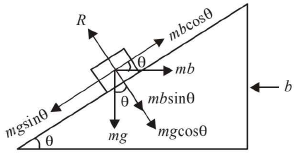mgcosθ - mbcosθ = ma
∴ a = (gsinθ - bcosθ)
If a = 0, b = gtanθ.
Offer running on EduRev: Apply code STAYHOME200 to get INR 200 off on our premium plan EduRev Infinity!

,

,

,

,

,

,

,

,

,

,

,

,

,

,

,

,

,

,

,

,

,

;